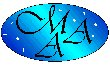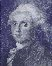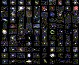## NGC and IC numbers associated with Messier Objects

There are four Messier objects which have no NGC number assigned: M25 (which is IC 4725), the double star M40, the Pleiades M45 (some of their nebula have NGC numbers, however) and the Milky Way Patch M24 (which contains open cluster NGC 6603 though, and may be identical to IC 4715).

All other Messier objects were assigned with NGC numbers also, and in some cases, parts of them got additional numbers, so that not every NGC or IC entry is visible from the Messier List "sorted by Right Ascension". Here we give a hopefully complete list of NGC and IC numbers related to Messier objects. Please submit any corrections, possible omissions, or comments !

Also look at our Messier Object Cross Reference list with other catalog assignments of Messier's objects.

### NGC numbers related to Messier objects

```N 205 =  Gx M110
N 206 = C+N in Gx  M31
N 221 =  Gx  M32
N 224 =  Gx  M31
N 588 =  Kt in Gx  M33
N 581 =  OC M103
N 592 =  Kt in Gx  M33
N 595 =  Kt in Gx  M33
N 598 =  Gx  M33
N 603 = *** in Gx  M33
N 604 =  Kt in Gx  M33
N 628 =  Gx  M74
N 650 = Pt. of Pl  M76 (South Preceding/W)
N 651 = Pt. of Pl  M76 (H I.193, North Following/E)
N1039 =  OC  M34
N1068 =  Gx  M77
N1432 =  Nb in OC  M45 (Maia Neb. in Pleiades)
N1435 =  Nb in OC  M45 (Merope Neb. in Pleiades)
N1904 =  Gb  M79
N1922 =  OC  M38
N1952 = SNR   M1
N1976 =  Nb  M42
N1982 =  Nb  M43
N1960 =  OC  M36
N2068 =  Nb  M78
N2099 =  OC  M37
N2168 =  OC  M35
N2287 =  OC  M41
N2323 =  OC  M50
N2422 =  OC  M47
N2437 =  OC  M46
N2438 =  PN superimposed on OC  M46
N2447 =  OC  M93
N2478 = erroneous position for  M47, no object
N2548 =  OC  M48
N2632 =  OC  M44
N2682 =  OC  M67
N3031 =  Gx  M81
N3034 =  Gx  M82
N3351 =  Gx  M95
N3368 =  Gx  M96
N3379 =  Gx M105
N3556 =  Gx M108
N3587 =  Pl  M97
N3623 =  Gx  M65
N3627 =  Gx  M66
N3953 =  Gx M109B, discovered by Méchain and probably observed by Messier
N3992 =  Gx M109, probably discovered and observed by Messier
N4192 =  Gx  M98
N4254 =  Gx  M99
N4258 =  Gx M106
N4303 =  Gx  M61
N4321 =  Gx M100
N4374 =  Gx  M84
N4382 =  Gx  M85
N4406 =  Gx  M86
N4472 =  Gx  M49
N4486 =  Gx  M87
N4501 =  Gx  M88
N4548 =  Gx  M91
N4552 =  Gx  M89
N4569 =  Gx  M90
N4571 =  Gx; erroneous "M91?" in NGC (and J.H.'s GC )
N4579 =  Gx  M58
N4590 =  Gb  M68
N4594 =  Gx M104
N4621 =  Gx  M59
N4649 =  Gx  M60
N4736 =  Gx  M94
N4826 =  Gx  M64
N5024 =  Gb  M53
N5055 =  Gx  M63
N5194 =  Gx  M51
N5195 =  Gx  M51B; close companion of M51, discovered by Méchain and described by Messier
N5236 =  Gx  M83
N5272 =  Gb   M3
N5447 =  Kt in Gx M101 (H III.787)
N5449 =  Kt in Gx M101
N5450 =  Kt in Gx M101
N5451 =  Kt in Gx M101
N5453 =  Kt in Gx M101
N5455 =  Kt in Gx M101
N5457 =  Gx M101
N5458 =  Kt in Gx M101 (ne in NGC 2000.0)
N5461 =  Kt in Gx M101 (H III.788)
N5462 =  Kt in Gx M101 (H III.789)
N5866 =  Gx M102
N5904 =  Gb   M5
N6093 =  Gb  M80
N6121 =  Gb   M4
N6171 =  Gb M107
N6205 =  Gb  M13
N6218 =  Gb  M12
N6254 =  Gb  M10
N6266 =  Gb  M62
N6273 =  Gb  M19
N6341 =  Gb  M92
N6333 =  Gb   M9
N6402 =  Gb  M14
N6405 =  OC   M6
N6475 =  OC   M7
N6494 =  OC  M23
N6514 =  Nb  M20
N6523 =  Nb   M8
N6526 =  Nb, Pt. of   M8
N6530 =  OC, Pt. of   M8
N6531 =  OC  M21
N6533 =  Nb, Pt. of   M8
N6603 =  OC in MWP  M24
N6611 = C+N  M16
N6613 =  OC  M18
N6618 =  Nb  M17
N6626 =  Gb  M28
N6637 =  Gb  M69
N6656 =  Gb  M22
N6681 =  Gb  M70
N6694 =  OC  M26
N6705 =  OC  M11
N6720 =  Pl  M57
N6715 =  Gb  M54
N6779 =  Gb  M56
N6809 =  Gb  M55
N6838 =  Gb  M71
N6853 =  Pl  M27
N6864 =  Gb  M75
N6913 =  OC  M29
N6981 =  Gb  M72
N6994 =  4*  M73
N7078 =  Gb  M15
N7092 =  OC  M39
N7089 =  Gb   M2
N7099 =  Gb  M30
N7654 =  OC  M52
```

### IC numbers related to Messier objects

• IC I
```I 131 =  Nb in Gx  M33
I 132 =  Nb in Gx  M33
I 133 =  Nb in Gx  M33
I 134 =   * in Gx  M33
I 135 =  Nb in Gx  M33
I 136 =  Nb in Gx  M33
I 137 =  Nb in Gx  M33
I 139 =   * in Gx  M33
I 140 =   * in Gx  M33
I 142 =  Nb in Gx  M33
I 143 =  Nb in Gx  M33
I 349 =  Nb in OC  M45 (Pt. of Merope Neb. in M45)
I 353 =  Nb near OC  M45
```
• IC II
```I1990 =  ? object near OC  M45 ?
I4703 =  Nb  M16
I4715 =  MWP  M24
I4725 =  OC  M25
```

### Messier Objects not assigned NGC or IC numbers

```    - =  Star Cloud  M24 (Milky Way Patch; no number but contains NGC 6603; may be identical to IC 4715)
- =  Double Star  M40 (Winnecke 4)
- =  OC  M45 (the Pleiades have no NGC or IC number)
```

### Key

for the types given in above table
```  C+N = (Open) Cluster and (Diffuse) Nebula
Gb  = Globular Cluster
Gx  = Galaxy
Kt  = Knot
MWP = Milky Way Patch
Nb  = (Diffuse) Nebula
OC  = Open Cluster
Pt  = Part
SNR = Supernova Remnant
*   = Star (or starlike object)
*** = 3 starlike objects
4*  = 4 stars
```

Hartmut Frommert
Christine Kronberg
[contact]Last Modification: April 26, 2013